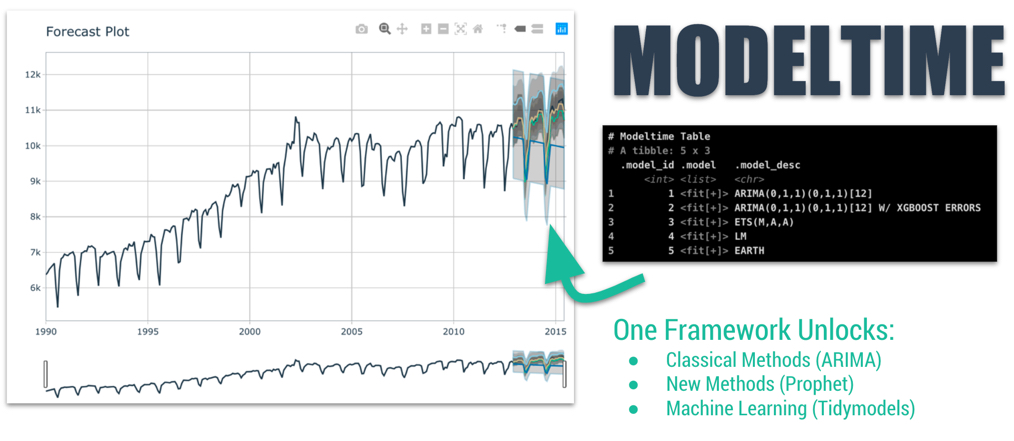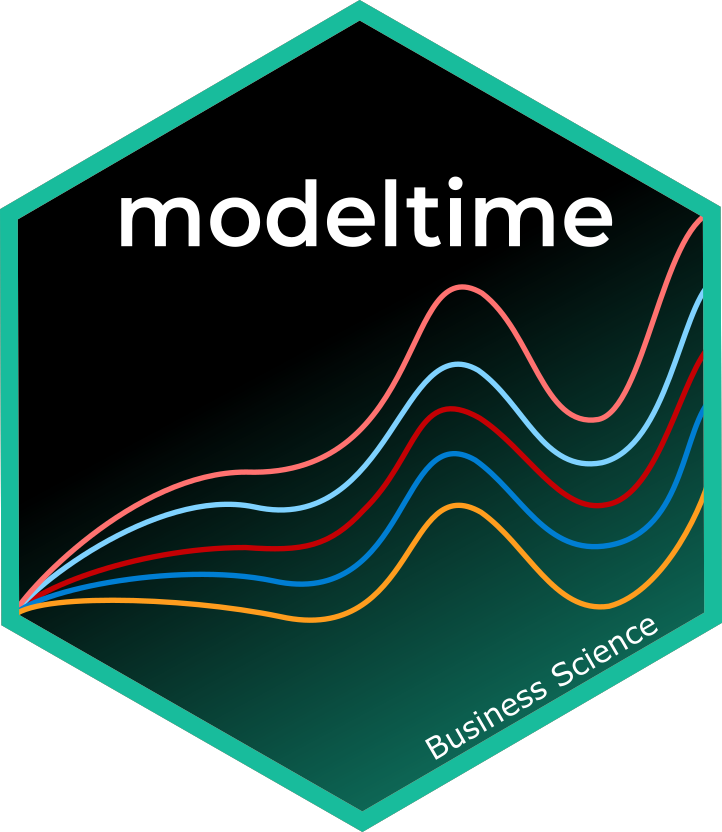# Getting Started with ModeltimeForecasting with `tidymodels` made easy! This short tutorial shows how you can use:

• Modeltime models like `arima_reg()`, `arima_boost()`, `exp_smoothing()`, `prophet_reg()`, `prophet_boost()`, and more
• Parsnip models like `linear_reg()`, `mars()`, `svm_rbf()`, `rand_forest()`, `boost_tree()` and more

To perform classical time series analysis and machine learning in one framework! See “Model List” for the full list of `modeltime` models.

## The Modeltime Workflow

Here’s the general process and where the functions fit.